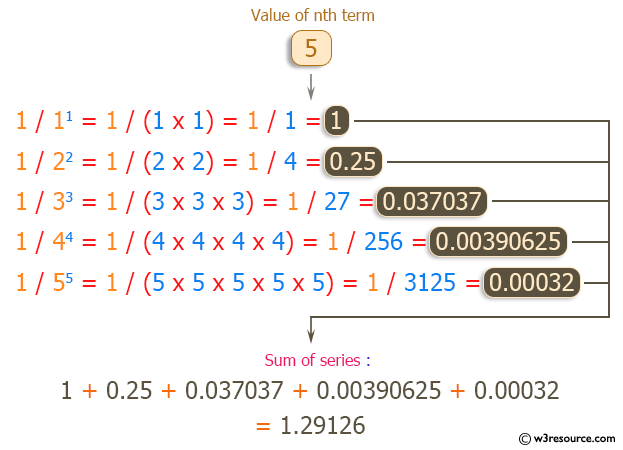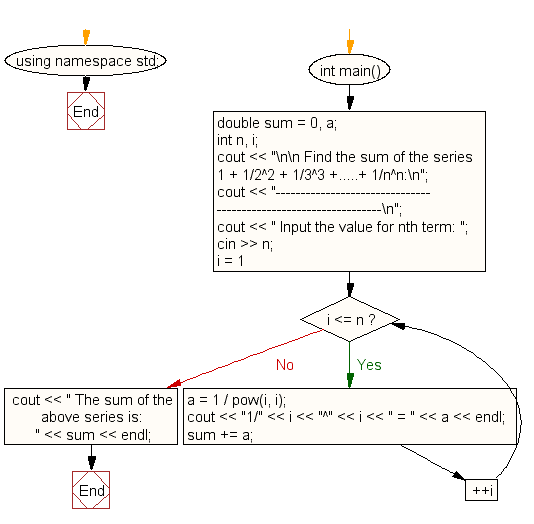﻿ C++ : Find the sum of the series 1+ 1/2^2 + 1/3^3 +..+ 1/n^n# C++ Exercises: Find the sum of the series 1 + 1/2^2 + 1/3^3 + …..+ 1/n^n

## C++ For Loop: Exercise-11 with Solution

Write a program in C++ to find the sum of the series 1 + 1/2^2 + 1/3^3 + …..+ 1/n^n.

Pictorial Presentation:Sample Solution :-

C++ Code :

``````#include <iostream>
#include <math.h>
using namespace std;

int main()
{
double sum = 0, a;
int n, i;
cout << "\n\n Find the sum of the series 1 + 1/2^2 + 1/3^3 +.....+ 1/n^n:\n";
cout << "----------------------------------------------------------------\n";
cout << " Input the value for nth term: ";
cin >> n;
for (i = 1; i <= n; ++i)
{
a = 1 / pow(i, i);
cout << "1/" << i << "^" << i << " = " << a << endl;
sum += a;
}
cout << " The sum of the above series is: " << sum << endl;
}
``````

Sample Output:

``` Find the sum of the series 1 + 1/2^2 + 1/3^3 +.....+ 1/n^n:
----------------------------------------------------------------
Input the value for nth term: 5
1/1^1 = 1
1/2^2 = 0.25
1/3^3 = 0.037037
1/4^4 = 0.00390625
1/5^5 = 0.00032
The sum of the above series is: 1.29126
```

Flowchart:C++ Code Editor:

Contribute your code and comments through Disqus.

What is the difficulty level of this exercise?

﻿

## C++ Programming: Tips of the Day

What is a smart pointer and when should I use one?

This answer is rather old, and so describes what was 'good' at the time, which was smart pointers provided by the Boost library. Since C++11, the standard library has provided sufficient smart pointers types, and so you should favour the use of std::unique_ptr, std::shared_ptr and std::weak_ptr.

There was also std::auto_ptr. It was very much like a scoped pointer, except that it also had the "special" dangerous ability to be copied - which also unexpectedly transfers ownership.

It was deprecated in C++11 and removed in C++17, so you shouldn't use it.

```std::auto_ptr<MyObject> p1 (new MyObject());
std::auto_ptr<MyObject> p2 = p1; // Copy and transfer ownership.
// p1 gets set to empty!
p2->DoSomething(); // Works.
p1->DoSomething(); // Oh oh. Hopefully raises some NULL pointer exception.
```

Ref : https://bit.ly/3mc9GHE